# AES加密算法¶

## 一、简介¶

AES 算法用来替代原先的DES$$Data Encryption Standard$$ ），已经被多方分析且广为全世界所使用。经过五年的甄选流程，高级加密标准由美国国家标准与技术研究院 （NIST）于 $$2001$$$$11$$$$26$$ 日发布于 $$FIPS PUB 197$$ ，并在 $$2002$$$$5$$$$26$$ 日成为有效的标准。 $$2006$$ 年，高级加密标准已然成为对称密钥加密中最流行的算法之一 。 该算法为比利时密码学家 $$Joan Daemen和Vincent Rijmen$$ 所设计，结合两位作者的名字，以 $$Rijdael$$ 之名命之，投稿高级加密标准的甄选流程。（ $$Rijdael$$ 的发音近于 " $$Rhine doll$$ "。）

## 二、原理¶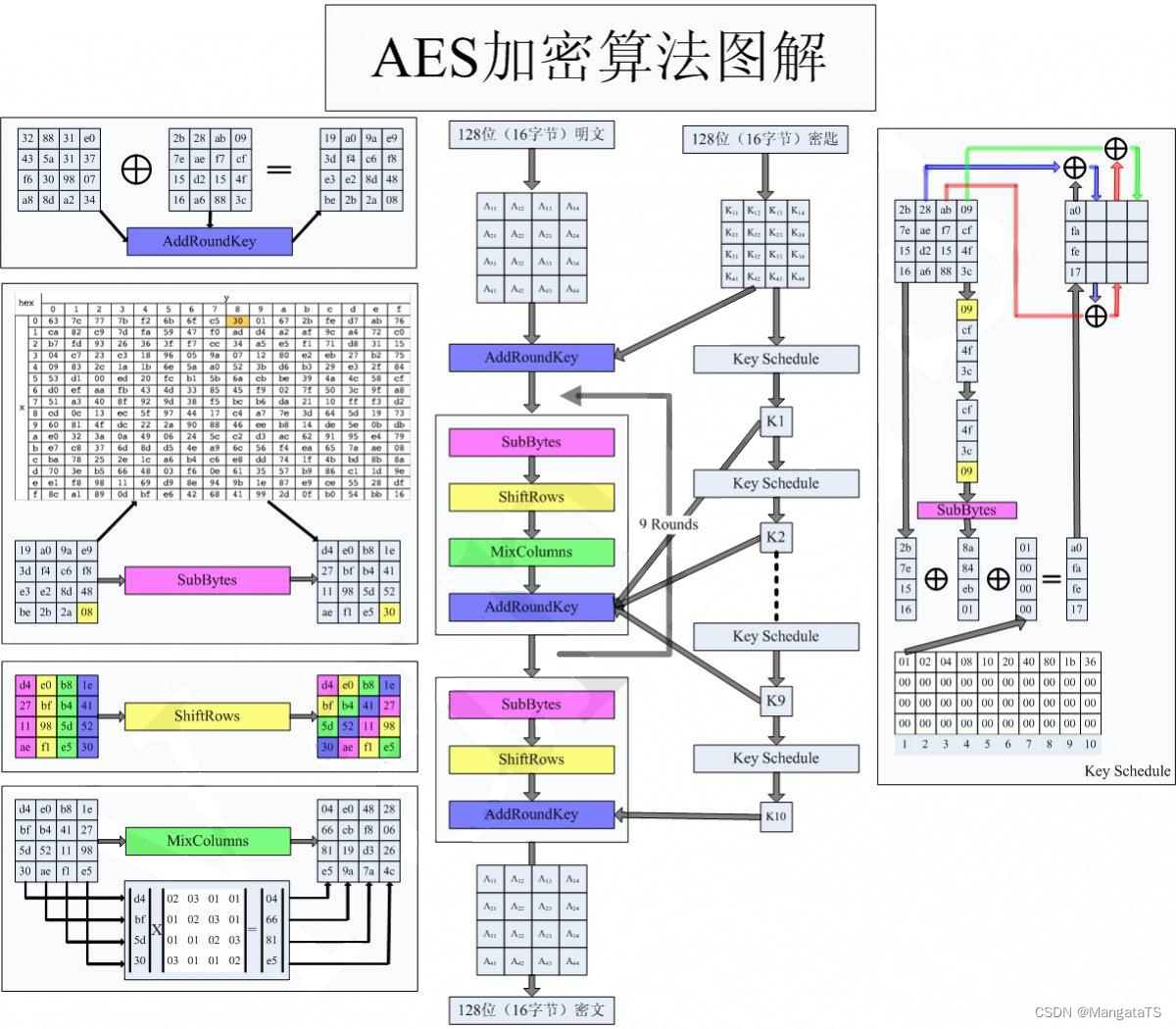### 2.1 明文加密流程¶

• 初始变换（其实就是 轮密钥加 操作）
• 九轮轮函数操作
• 字节代换（ $$SubBytes$$
• 行位移（ $$ShiftRows$$
• 列混淆 （ $$MixColumns$$
• 轮密钥加（ $$AddRoundKey$$
• 最终轮运算（实际上是 除去 列混淆的轮操作函数）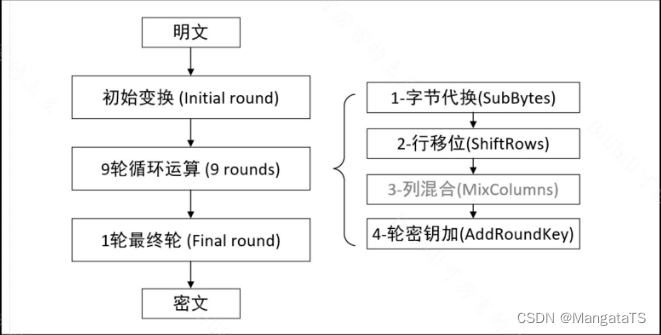### 2.2 子密钥生成流程¶

• 如果当前拓展的列 $$i$$ 不是 $$4$$ 的倍数，那么第 $$i$$ 列由如下等式确定：（其中 $$W[i]$$ 表示的就是第 $$i$$ 列的数据）
$W[i]=W[i-4] \ Xor \ W[i-1]$
• 如果当前拓展的列 $$i$$$$4$$ 的倍数，那么第 $$i$$ 列由如下等式确定：
$W[i]=W[i-4] \ Xor \ T(W[i-1])$

### 2.3 字节代换¶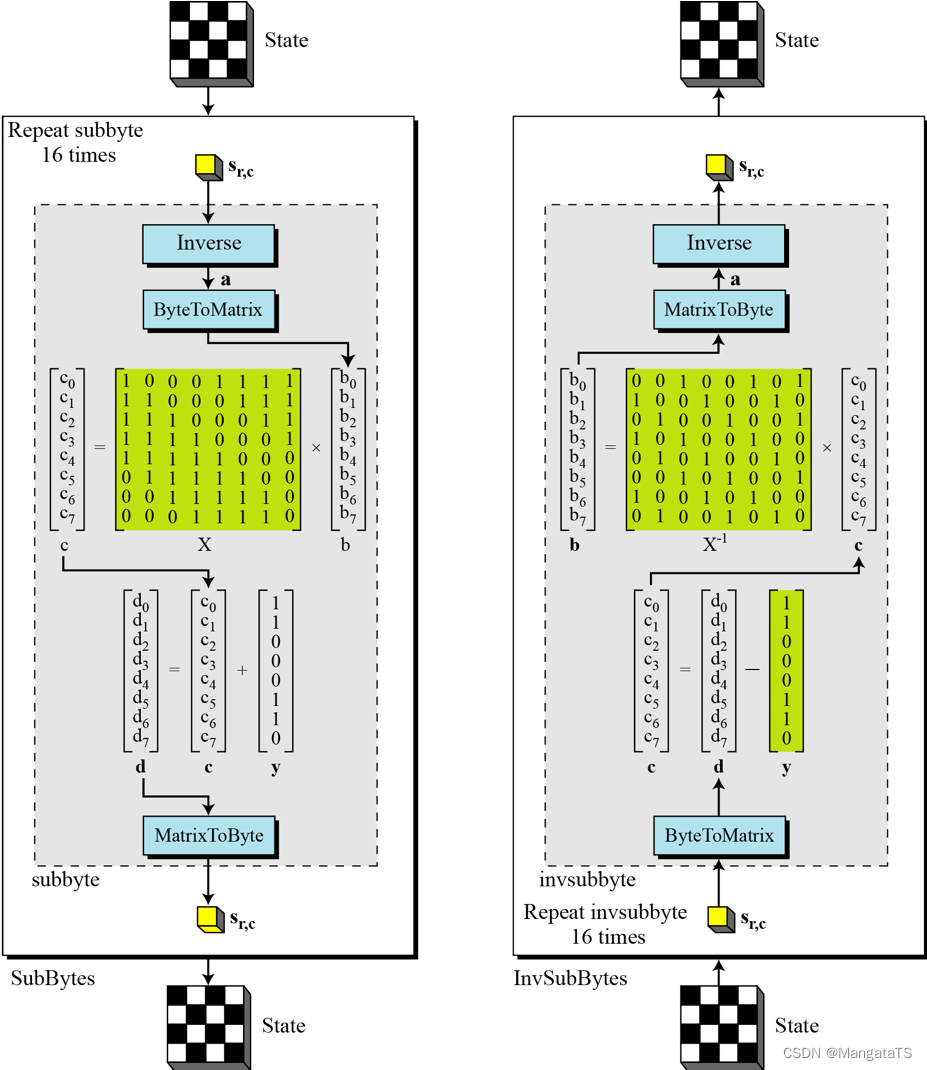### 2.4 行位移¶

• 对于第一行，我们保持不变
• 对于第二行，我们向左循环移动 $$1$$ 个字节
• 对于第三行，我们向左循环移动 $$2$$ 个字节
• 对于第四行，我们向左循环移动 $$3$$ 个字节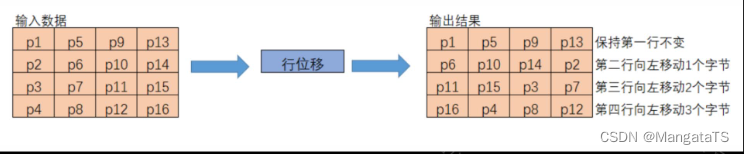### 2.5 列混淆（混合）¶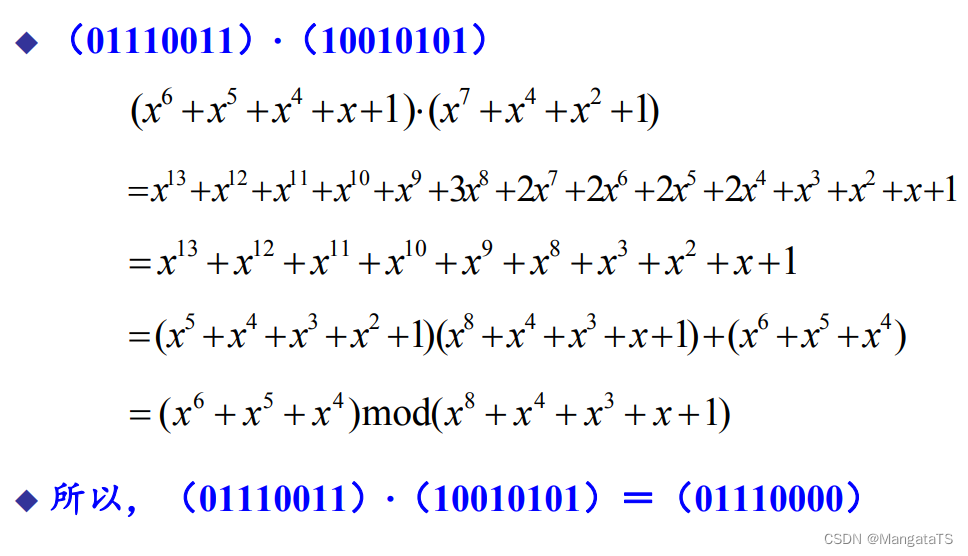然后加法变成了异或运算，例如，我们现在左边矩阵第一行的元素都是 $$a_i$$ ，右边的矩阵元素都是 $$b_i$$ ，顺序如下：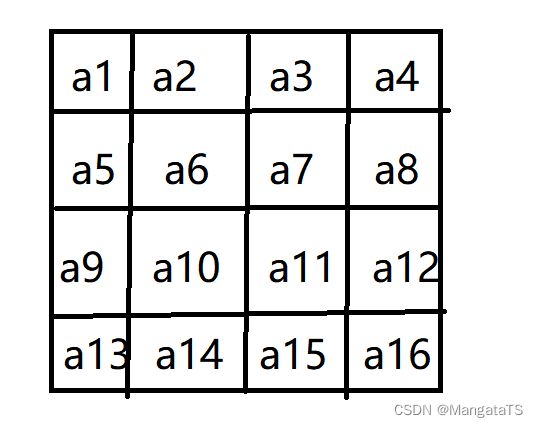B矩阵同理，那么我们想算出新矩阵 $$C$$ 的第一个字节的值就为：

$c_1 = (a_1 \times b_1) ⊕ (a_2 \times b_5) ⊕ (a_3 \times b_9) ⊕ (a_4 \times a_{13})$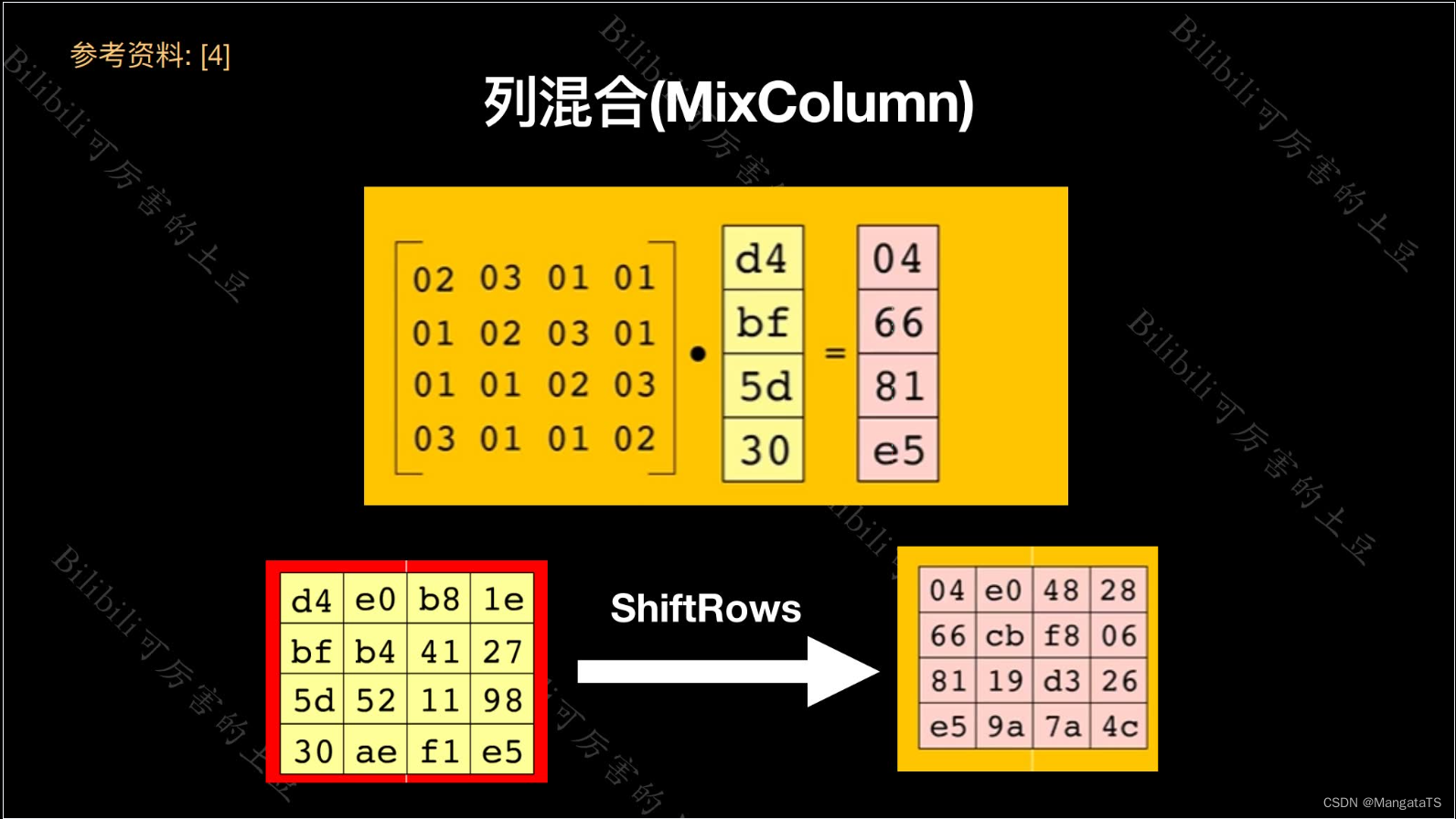### 2.6 轮密钥加变换¶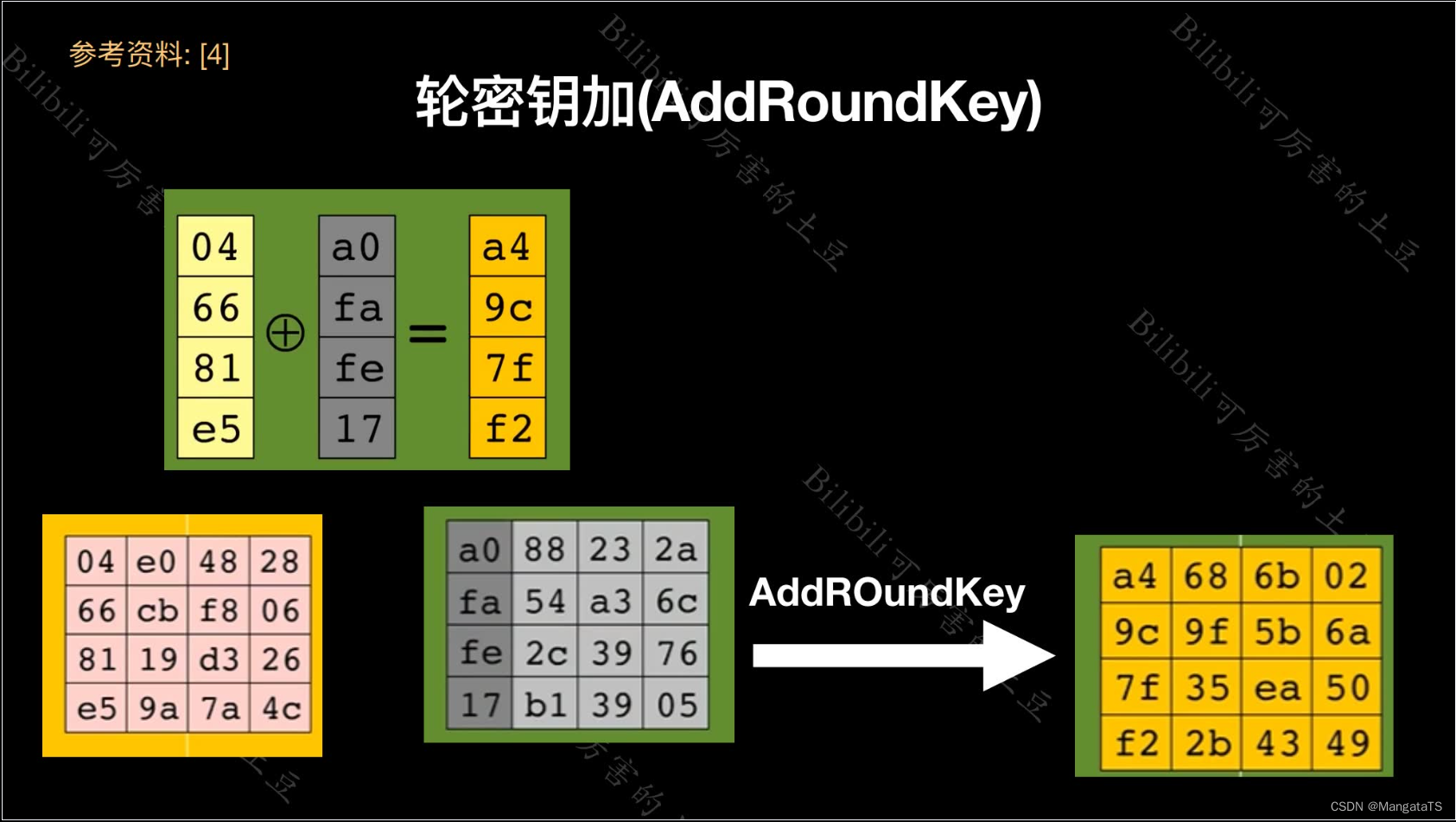### 2.7 密钥拓展中的T函数¶

$$T$$ 函数由三个部分组成： 字循环字节代换轮常量异或

#### 2.7.1 字循环¶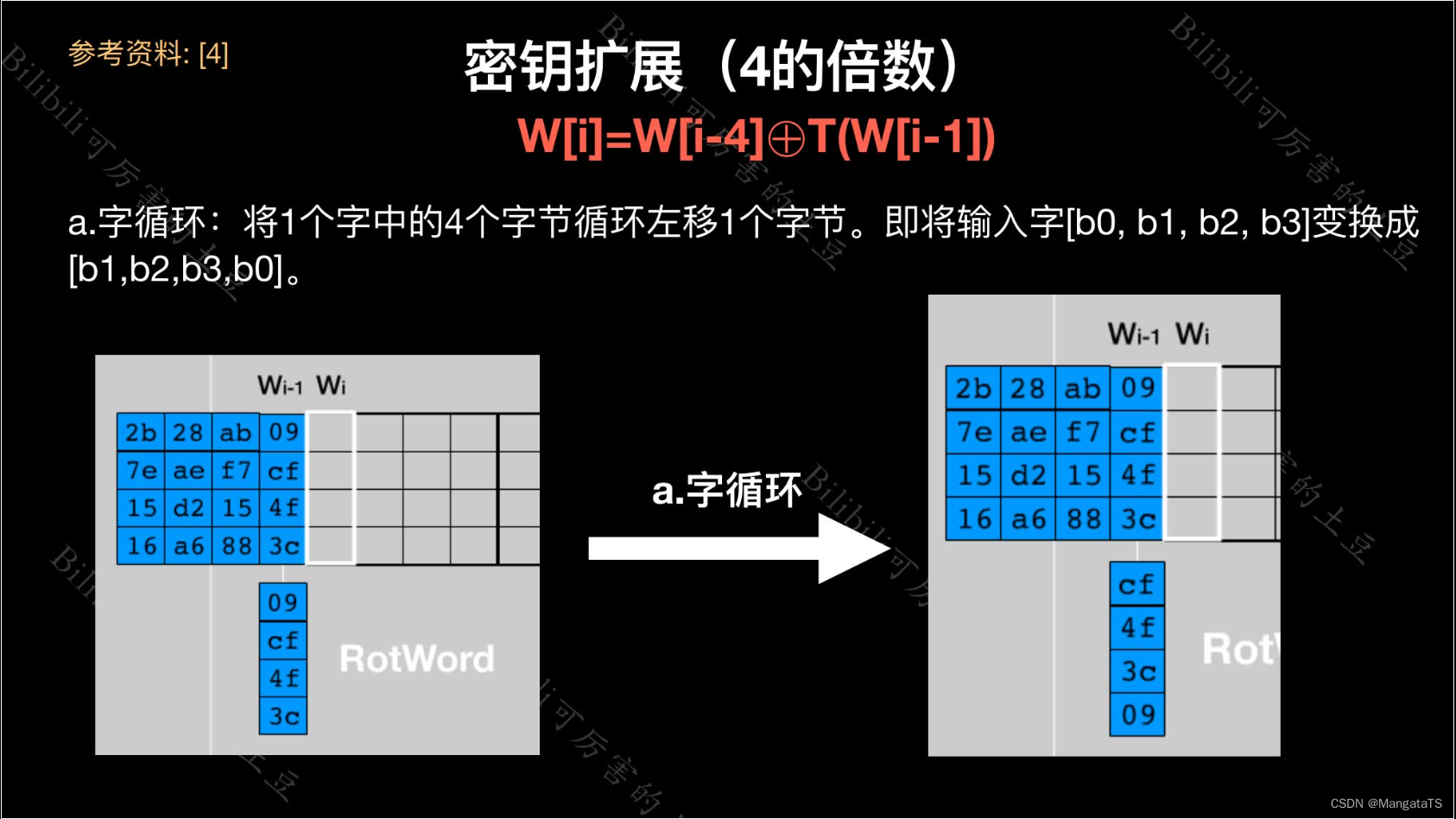### 2.7.3 轮常量异或¶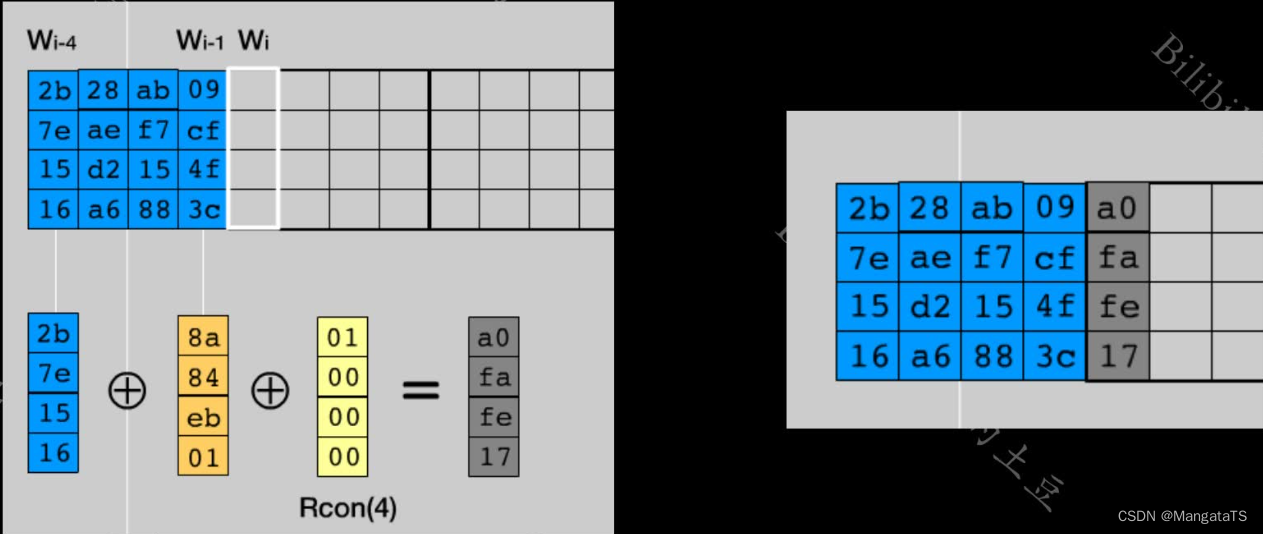## 三、优缺点¶

### 3.1 优点¶

• 安全性高，稳定的数学基础、没有算法弱点、算法抗密码分析强度高
• 性能好，能在多个平台上以较快的速度实现
• 存储空间小，不能占用大量的存储空间和内存
• 灵活性、硬件、软件适应性、算法的简单性等
• 很好的抵抗差分密码分析及线性密码分析的能力。
• AES的密钥长度比DES大，它也可设定为32比特的任意倍数，最小值为128比特，最大值为256比特，所以用穷举法是不可能破解的。

## 四、对比DES¶

### 4.1 相同之处¶

• 两者的轮函数都是由三部分构成，非线性层、线性混合层、子密钥异或，只不过顺序不同
• AES的子密钥异或对应于DES$$S$$ 盒子之前的子密钥异或
• AES的列混淆运算的目的是为了让不同的字节相互影响，而DESF函数的输出于左边一半数据相加也有类似的效果
• AES的非线性运算是字节代替（ByteSub），对应于DES中的非线性运算 $$S$$ 盒子
• 行位移运算保证了每一行的字节不仅仅影响其他行对应的字节，而且影响其他行所有的字节，这与 DES 中的置换 $$IP$$ 相似

### 4.2 不同之处¶

• AES 的密钥长度（ $$128$$ 位、 $$192$$ 位、 $$256$$ 位）是可变的，而 DES 的密钥固定长度为 $$56$$
• DES 是面向比特运算的，而 AES 是面向字节运算的
• AES的加密运算和解密运算不一致，因而加密器不能同时作为解密器，而DES则无此限制